# Expressions and Keys

## Mathematical and Logical Expressions

Neper handles mathematical expressions thanks to the muparser library. The expression must contain no space, tabulation or new-line characters, and match the following syntax. 1

### Functions

The following table gives an overview of the functions supported by the default implementation. It lists the function names, the number of arguments and a brief description.

 Name Description sin sine function cos cosine function tan tangens function asin arcus sine function acos arcus cosine function atan arcus tangens function sinh hyperbolic sine function cosh hyperbolic cosine tanh hyperbolic tangens function asinh hyperbolic arcus sine function acosh hyperbolic arcus tangens function atanh hyperbolic arcur tangens function log2 logarithm to the base 2 log10 logarithm to the base 10 log logarithm to the base 10 ln logarithm to base $$e$$ (2.71828…) exp e raised to the power of x sqrt square root of a value sign sign function: -1 if $$x<0$$; 1 if $$x>0$$ rint round to nearest integer abs absolute value min min of all arguments max max of all arguments sum sum of all arguments avg mean value of all arguments

### Binary Operators

The following table lists the default binary operators supported by the parser.

 Operator Description Priority && logical and 1 || logical or 2 <= less or equal 4 >= greater or equal 4 != not equal 4 == equal 4 > greater than 4 < less than 4 + addition 5 - subtraction 5 * multiplication 6 / division 6 ^ raise x to the power of y 7

### Ternary Operators

The parser has built in support for the if-then-else operator. It uses lazy evaluation in order to make sure only the necessary branch of the expression is evaluated.

 Operator Description ?: if-then-else operator, following the C/C++ syntax: ()?:.

### Statistical Distributions

The following table lists the statistical distributions. Custom endpoints (not indicated) can also be added as arguments, as described in the following.

 Operator Description Information normal(,) normal lognormal(,) lognormal dirac() Dirac beta(,) beta function $$x>0$$, $$y>0$$ lorentzian(,) Lorentzian studentst(,) Student’s t weibull(k,) Weibull $$k > 0$$ represents the shape breitwigner(,[,]) Breit-Wigner $$\geq 0$$, default 1 expnormal(,[,]) exp-normal $$> 0$$, default  moffat(,[,]) Moffat $$> 0$$, default 1 pearson7(,[,]) Pearson type VII default $$= 1.5$$ pseudovoigt(,[,]) Pseudo-Voigt $$\in [0,\,1]$$, default 0.5 skewnormal(,[,]) skewed normal default $$=$$ custom() custom

mean represents the mean (or centre), and sigma represents the standard deviation (or scale, $$> 0$$). gamma depends on the distribution function (see the above table). For all distributions, custom endpoints can be added as last arguments, as <from_value>,<to_value>, where from_value is the lower endpoint and to_value is the upper endpoint. The parameter keywords do not need to be provided, but, when they are, the parameters can be given in any order, as in moffat(gamma=1,from=0,to=1,sigma=0.1,mean=0.5). Endpoints are considered inclusive by default, but exclusive endpoints can be specified using fromexclusive=<from_value> and toexclusive=<to_value> (frominclusive=<from_value> and toinclusive=<to_value> can be used for inclusive endpoints). String completion is available for the keywords. Finally, a sum of distributions of increasing averages can be provided, as in 0.3*lognormal(0.5,0.1)+0.7*normal(1,0.1).

In the case of the custom distribution, the numerical distribution must be provided in the file. The file must contain the x and y values of the distribution on successive lines. The x values must be provided in ascending order and form a regular grid. The distribution must contain at least 3 points and does not need to integrate to 1.

## Tessellation Keys

Available keys for a tessellation itself are provided below.

 Key Descriptor Apply to dim dimension tess vernb number of vertices tess edgenb number of edges tess facenb number of faces tess polynb number of polyhedra tess cellnb number of cells tess x x coordinate tess y y coordinate tess z z coordinate tess coo x, y and z coordinates tess area surface area tess vol volume tess size size (surface area/volume in 2D/3D) tess

Available keys for tessellation seeds, vertices, edges, faces, polyhedra, crystals and cell groups are provided below. Also note that the keys apply to cells if they are tagged to apply to polyhedra and the tessellation is 3D and faces and the tessellation is 2D, and that keys apply to crystals if they apply to cells. You may also replace, in the tessellation keys themselves, poly by cell if the tessellation is 3D and face by cell if the tessellation is 2D (it applies only in rare cases). For example, for a 2D tessellation, you may use -statcell ncells instead of -statface nfaces. Keys specific to cells are defined accordingly in the following but also apply to polys is the tessellation is 3D and faces is the tessellations is 2D.

To turn a key value into a value relative to the mean over all entities (e.g. the relative cell size), append the key expression with the :rel modifier. To turn a key value into a value which holds for a unit cell size, append the key expression with the :uc modifier. To use as a reference only the body or true entities (see below), append b or t to the modifiers, respectively.

 Key Descriptor Apply to id identifier seed, ver, edge, face, poly, group x x coordinate seed, ver, edge, face, poly y y coordinate seed, ver, edge, face, poly z z coordinate seed, ver, edge, face, poly coo x, y and z coordinates seed, ver, edge, face, poly xmin minimum x coordinate edge, face, poly ymin minimum y coordinate edge, face, poly zmin minimum z coordinate edge, face, poly xmax maximum x coordinate edge, face, poly ymax maximum y coordinate edge, face, poly zmax maximum z coordinate edge, face, poly w weight (width for a lamellar tessellation) seed, cell true true level ver, edge, face, poly body body level ver, edge, face, poly state state ver, edge, face, poly domtype type of domain (0 if on a domain vertex, 1 if on a domain edge and 2 if on a domain face) ver, edge, face domface domain face (-1 if undefined) face domedge domain edge (-1 if undefined) edge domver domain vertex (-1 if undefined) ver scale scale face 2 length length edge area surface area face, poly, group vol volume poly, group size size (surface area/volume in 2D/3D) cell, group diameq equivalent diameter 3 face, poly avdiameq average equivalent diameter 3 face, poly radeq equivalent radius (half of the eq. diameter) face, poly avradeq average equivalent radius (half of the eq. diameter) face, poly sphericity sphericity 4 poly circularity circularity 5 face convexity convexity 6 face (only for a 2D tessellation), poly dihangleav average dihedral angle face, poly dihanglemin minimum dihedral angle face, poly dihanglemax maximum dihedral angle face, poly dihangles dihedral angles face, poly ff flatness fault (in degrees) face theta disorientation angle (in degrees) edge (in 2D), face (in 3D) cyl cylinder polygonization 18 edge vernb number of vertices edge, face, poly vers vertices edge, face, poly edgenb number of edges ver, face, poly edges edges ver, face, poly facenb number of faces ver, edge, poly faces faces ver, edge, poly polynb number of polyhedra ver, edge, face polys polyhedra ver, edge, face nfacenb number of neighboring faces face nfaces neighboring faces face nfacenb_samedomain number of neighboring faces of the same domain (parent cell of a multiscale tessellation) face (in 2D) nfaces_samedomain neighboring faces of the same domain (parent cell of a multiscale tessellation) face (in 2D) npolynb number of neighboring polyhedra poly npolys neighboring polyhedra poly npolys_unsort neighboring polyhedra, unsorted list poly npolynb_samedomain number of neighboring polyhedra of the same domain (parent cell of a multiscale tessellation) poly npolys_samedomain neighboring polyhedra of the same domain (parent cell of a multiscale tessellation) poly vercoos vertex coordinates face, poly faceareas face surface areas poly faceeqs face equations 8 poly nseednb number of neighboring seeds poly nseeds neighboring seeds 9 poly scaleid() identifier of the corresponding cell at scale  cell lam lamella width id 10 cell mode mode 11 cell group group cell per periodic (1 if periodic, 0 otherwise) ver, edge, face (in 3D) fiber(...) 1 if in orientation fiber and 0 otherwise, see Orientation Fibers poly  orientation descriptor face (in 2D), poly (in 3D)

Variables consisting of several values (vers, etc.) are not available for sorting (option -sort).

For a cell, the body and true variables are defined as follows,

• body is an integer equal to 0 if the cell is at the domain boundary, i.e. if it shares at least one face with it (edge in 2D), and is equal to 1 or higher otherwise. This is determined as follows: if a cell is surrounded by cells with body values equal to or higher than n, its body value is equal to n + 1. Therefore, body tends to increase with the distance to the domain boundary and can be used to define cells that may suffer from boundary effects.

• true is an integer equal to 0 it the cell shape is biased by the domain boundary, and is equal to 1 or higher otherwise. A value higher than 0 is achieved if and only if any seed that would have been located outside the domain (where it could not be) would not have affected the shape of the cell. This condition is fulfilled if the distance between the seed of the cell and any of its vertices is lower than the minimum distance between a vertex of the cell and the domain boundary. true is extended to values higher than 1 in the same way as body: if a cell is surrounded by cells with true values equal to or higher than n, its true value is equal to n + 1. As body, true tends to increase with the distance to the domain boundary, and $$true \leq body$$. true is especially useful for statistics on the cells (morphology, mesh, etc.), for which only cells with $$true \geq 1$$ should be considered.

For entities of lower dimension than cells (vertices, edges and faces), body and true are equal to the maximum bodyor :data:true values of the cells they belong to.

## Raster Tessellation Keys

Available keys for raster tessellation itself are provided below.

 Key Descriptor Apply to dim dimension tesr voxnbx number of voxels in direction x tesr voxnby number of voxels in direction y tesr voxnbz number of voxels in direction z tesr voxnb number of voxels in total tesr originx origin x coordinate tesr originy origin y coordinate tesr originz origin z coordinate tesr voxsizex voxel size in direction x tesr voxsizey voxel size in direction y tesr voxsizez voxel size in direction z tesr rastersizex raster size in direction x tesr rastersizey raster size in direction y tesr rastersizez raster size in direction z tesr rastersize raster size (surface area/volume in 2D/3D) tesr area surface area tesr vol volume tesr size size (surface area/volume in 2D/3D) tesr x x coordinate tesr y y coordinate tesr z z coordinate tesr coo x, y and z coordinates tesr

Available keys for raster tessellation seeds, cells, cell groups and voxels are provided below. Mathematical and logical expressions based on these keys can also be used. To turn a key value into a value relative to the mean over all entities (e.g.the relative cell size), append the key expression with the :rel modifier. To turn a key value into a value which holds for a unit cell size, append the key expression with the :uc modifier.

### General

 Key Descriptor Applies to id identifier seed, cell, group, voxel cell cell voxel oridef orientation is defined voxel w Laguerre weight seed

### Geometry

 Key Descriptor Applies to x x coordinate seed, cell, voxel y y coordinate seed, cell, voxel z z coordinate seed, cell, voxel coo x, y and z coordinates seed, cell, voxel vx x coordinate (in voxel) voxel vy y coordinate (in voxel) voxel vz z coordinate (in voxel) voxel vcoo x, y and z coordinates (in voxel) voxel vxmin minimum x coordinate (in voxel) cell vymin minimum y coordinate (in voxel) cell vzmin minimum z coordinate (in voxel) cell vxmax maximum x coordinate (in voxel) cell vymax maximum y coordinate (in voxel) cell vzmax maximum z coordinate (in voxel) cell domvxmin domain minimum x coordinate (in voxel), always 1 domain domvymin domain minimum y coordinate (in voxel), always 1 domain domvzmin domain minimum z coordinate (in voxel), always 1 domain domvxmax domain maximum x coordinate (in voxel) domain domvymax domain maximum y coordinate (in voxel) domain domvzmax domain maximum z coordinate (in voxel) domain area surface area cell, group (in 2D) vol volume cell, group (in 3D) size size (surface area/volume in 2D/3D) cell, group areafrac surface area fraction group (in 2D) volfrac volume fraction group (in 3D) sizefrac size fraction (surface area/volume fraction in 2D/3D) group diameq equivalent diameter 3 cell radeq equivalent radius cell convexity convexity 6 cell

### Orientation

 Key Descriptor Applies to  orientation descriptor voxel, cell gos grain orientation spread 7 cell oridisanisoangles orientation distribution anisotropy / principal angles 12 cell oridisanisoaxes orientation distribution anisotropy / principal axes 12 cell oridisanisofact orientation distribution anisotropy factor 12 cell oridisanisodeltas orientation distribution anisotropy / principal delta angles 13 cell

## Tessellation Optimization Keys

### Time Keys

The available keys for option -morphooptilogtime are provided below. Use iter(<factor>), where factor is an integer reduction factor, to log values only at specific iteration numbers.

 Key Descriptor iter iteration number varupdateqty number of updated variables seedupdateqty number of updated seeds seedupdatelist list of updated seeds cellupdateqty number of updated cells cellupdatelist list of updated cells var time for variable update seed time for seed update cell_init time for cell update initialization cell_kdtree time for cell update kdtree computation cell_shift time for cell update shift computation cell_neigh time for cell update neighbor computation cell_cell time for cell update cell computation cell_other time for cell update others cell_total total time for cell update val time for (objective function) value update val_init time for (objective function) value update / initialization val_penalty time for (objective function) value update / penalty computation val_val time for (objective function) value update / value computation val_val_cellval time for (objective function) value update / value computation / cell values val_val_comp time for (objective function) value update / value computation / computation val_comp time for (objective function) value update / computation total total time cumtotal cumulative total time

### Variable Keys

The available keys for option -morphooptilogvar are provided below. Use iter(<factor>), where factor is an integer reduction factor, to log values only at specific iteration numbers.

 Key Descriptor Apply to iter iteration number n/a id identifier seed x x coordinate seed y y coordinate seed z z coordinate seed w weight seed

### Objective Function Value Keys

The available keys for option -morphooptilogval are provided below. Use iter(<factor>), where factor is an integer reduction factor, to log values only at specific iteration numbers.

 Key Descriptor iter iteration number val value valmin minimal value val0 value, without smoothing valmin0 minimal value, without smoothing val() i th subvalue val0() i th subvalue, without smoothing eps error on the objective function (see -morphooptistop) reps relative error on the objective function (see -morphooptistop) loop optimization loop plateaulength current plateau length 14

### Statistical Distribution Keys

The available keys for option -morphooptilogdis are provided below. PDF stands for probability density function and CDF stands for cumulative probability density function. Use iter(<factor>), where factor is a reduction factor, to log values only at specific iteration numbers.

 Key Descriptor iter iteration number x x coordinate tarpdf target PDF tarcdf target CDF curpdf current PDF curcdf current CDF tarpdf0 target PDF, not smoothed tarcdf0 target CDF, not smoothed curcdf0 current CDF, not smoothed

### Raster Tessellation Voxel Keys

The available keys for option -morphooptilogtesr are provided below. Values are written for each voxel used to compute the objective function. Use iter(<factor>), where factor is a reduction factor, to log values only at specific iteration numbers.

 Key Descriptor iter iteration number id cell identifier x x coordinate y y coordinate z z coordinate dist distance to the cell

## Orientation Optimization Keys

### Variable Keys

The available keys for option -orioptilogvar are provided below. For all orientation descriptors but quaternion, the returned orientation are located in the fundamental region. Use iter(<factor>), where factor is an integer reduction factor, to log values only at specific iteration numbers.

 Key Descriptor Apply to iter iteration number n/a id identifier seed rodrigues Rodrigues vector seed euler-bunge Euler angles (Bunge convention) seed euler-kocks Euler angles (Kocks convention) seed euler-roe Euler angles (Roe convention) seed rotmat Rotation matrix seed axis-angle rotation axis / angle pair seed quaternion quaternion seed

## Mesh Keys

Available keys for a mesh itself are provided below. “co” stands for “cohesive”.

 Key Descriptor Apply to eltnb element number {0-3}D,co mesh nodenb node number {0-3}D mesh elsetnb elset number {0-3}D,co mesh partnb partition number highest-dimension mesh x x coordinate {0-3}D mesh y y coordinate {0-3}D mesh z z coordinate {0-3}D mesh coo x, y and z coordinates {0-3}D mesh length length 1D mesh area surface area 2D mesh vol volume 3D mesh size size (length/area/volume in 1D/2D/3D) 1D mesh, 2D mesh, 3D mesh

Available keys for mesh node, elements and element sets (of all dimensions) and points are provided below. “co” stands for “cohesive”.

 Key Descriptor Apply to id identifier node, {0-3}D,co elt, {0-3}D,co elset x x coordinate node, {0-3}D,co elt, {0-3}D elset y y coordinate node, {0-3}D,co elt, {0-3}D elset z z coordinate node, {0-3}D,co elt, {0-3}D elset coo x, y and z coordinates node, {0-3}D,co elt, {0-3}D elset dim lowest parent elt dimension node elset0d 0D elset 0D elt elset1d 1D elset 1D elt elset2d 2D elset 2D elt elset3d 3D elset 3D elt elsetco Cohesive elset co elt part partition {0-3}D elt, node group group {0-3}D elt, {0-3}D elset scaleid() identifier of the corresponding tess cell at scale  2D elset, 3D elset cyl cylinder polygonization 18 1D elt, 1D elset vol volume 3D elt, 3D elset area surface area 2D elt diameq equivalent diameter {2,3}D elt, {2,3}D elset radeq equivalent radius {2,3}D elt, {2,3}D elset length average edge length {0-3}D elt, 1D elset lengths edge lengths 2D elt, 3D elt elsetvol elset volume 3D elt elsetarea elset area 2D elt elsetlength elset length 1D elt rr radius ratio 3D elt rrav average radius ratio 3D elset rrmin min radius ratio 3D elset rrmax max radius ratio 3D elset Osize Osize 3D elset eltnb number of elements {0-3}D,co elset elts elements {0-3}D,co elset nodenb number of nodes {0-3}D,co elset nodes nodes {0-3}D,co elset true true level {0-3}D elt, {0-3}D elset body body level {0-3}D elt, {0-3}D elset domtype type of domain 15 {0-2}D elt, {0-2}D elset 2dmeshp closest point of the 2D mesh node, 3D elt 2dmeshd distance to 2dmeshp node, 3D elt 2dmeshv vector to 2dmeshp node, 3D elt 2dmeshn outgoing normal vector at 2dmeshp node, 3D elt per periodic (1 if periodic, 0 otherwise) {0,1}D elt, 2D elt (in 3D), {0,1}D elset, 2D elset (in 3D) col_rodrigues color in Rodrigues vector convention 16 node col_stdtriangle color in IPF convention 17 node fiber(...) 19 1 if in orientation fiber and 0 otherwise 3D elt, 3D elset theta disorientation angle (in degrees) 1D elt and elset (in 2D), 2D elt and elset (in 3D)  orientation descriptor 2D elt (in 2D), 2D elset (in 2D), 3D elt (in 3D), 3D elset (in 3D)

Variables beginning with 2dmesh are only available for statistics (options beginning with -stat of module -M); for elements, they apply to the centroids.

## Point Keys

Available keys for points are provided below.

 Key Descriptor Apply to Require id identifier point x x coordinate point y y coordinate point z z coordinate point cell cell point tessellation elt containing element point mesh elset containing elset point mesh 2dmeshp coordinates of the closest point of the 2D mesh point 3D mesh 2dmeshd distance to 2dmeshp point 3D mesh 2dmeshv vector to 2dmeshp point 3D mesh 2dmeshn outgoing normal vector of the 2D mesh at 2dmeshp point 3D mesh

## Simulation Results

A result of a simulation directory can be invoked simply from its name. A component of a vectorial or tensorial result can be invoked by prefixing the component to the name, as in coo1, stress11, etc. For a symmetrical tensor (for which only 6 values are stored), t, both t<i><j> and t<j><i> are valid. The type of a result of the simulation directory is determined automatically. Elset and mesh results can be obtained from the element results, by averaging or other statistical treatments.

Available results / keys and concerning orientation distributions are provided below.

 Key Descriptor Apply to ori average orientation elset, mesh oridisanisoangles orientation distribution principal angles elset, mesh oridisanisoaxes orientation distribution principal axes elset, mesh oridisanisofact orientation distribution factor elset, mesh odf(theta=) ODF defined at elements, with theta the standard deviation of the kernel (in degrees, optional) mesh odfn(theta=) ODF defined at nodes, with theta the standard deviation of the kernel (in degrees, optional) mesh

## Rotations and Orientations

### Rotation and Orientation Descriptors

Rotations and orientations can be described using the following descriptors.

 Key Descriptor Number of parameters rodrigues Rodrigues vector 3 euler-bunge Euler angles (Bunge convention) 3 euler-kocks Euler angles (Kocks convention) 3 euler-roe Euler angles (Roe convention) 3 rotmat rotation matrix 9 axis-angle rotation axis / angle pair 4 quaternion quaternion 4

The convention can be added to the descriptor, either active or passive, as in rodrigues:active. When no convention is provided, active is assumed.

Some options can take parameter values as argument, in which case the orientation must be expressed as <descriptor>(<parameter1>,<parameters2>,...). An example is rodrigues(0.1,0.2,0.3).

### Ideal Orientations

Keys are available for ideal orientations (lowercased is accepted):

 Key Miller indices Cube $$(0\,0\,1)[1\,0\,0]$$ Goss $$(0\,1\,1)[1\,0\,0]$$ U $$(1\,0\,1)[\overline{1}\,0\,1]$$ 45NDCube $$(0\,0\,1)[1\,\overline{1}\,0]$$ S1 $$(1\,2\,3)[6\,3\,\overline{4}]$$ S2 $$(\overline{1}\,2\,3)[6\,\overline{3}\,4]$$ S3 $$(1\,\overline{2}\,3)[6\,\overline{3}\,\overline{4}]$$ S4 $$(\overline{1}\,\overline{2}\,3)[6\,3\,4]$$ Brass1 $$(1\,1\,0)[1\,\overline{1}\,2]$$ Brass2 $$(\overline{1}\,1\,0)[1\,1\,\overline{2}]$$ Copper1 $$(1\,1\,2)[1\,1\,\overline{1}]$$ Copper2 $$(\overline{1}\,1\,2)[1\,\overline{1}\,1]$$

When loading orientations from an external file, use file(<file_name>[,des=<descriptor>]) where the orientation descriptor is among those listed above and is rodrigues:active by default.

### Orientation Fibers

Orientation fibers are defined by a crystal direction being parallel to a sample direction. Depending on the context, an angular tolerance or distribution with respect to the theoretical fiber can also be defined:

• fiber(<dirc_x>,<dirc_y>,<dirc_z>,<dirs_x>,<dirs_y>,<dirs_z>), where (<dirc_x>, <dirc_y>, <dirc_z>) is the crystal direction and (<dirs_x>, <dirs_y>, <dirs_z>) is the sample direction, corresponds to an ideal orientation fiber;

• fiber(<dirc_x>,<dirc_y>,<dirc_z>,<dirs_x>,<dirs_y>,<dirs_z>,<theta>), where <theta> is an angle expressed in degrees, corresponds to an orientation fiber with the angular tolerance <theta> from the ideal fiber;

• fiber(<dirc_x>,<dirc_y>,<dirc_z>,<dirs_x>,<dirs_y>,<dirs_z>):normal(<var>=<val>), where <var> can be theta or thetam) and <val> is the value, corresponds to an orientation fiber with a normal (Gaussian) disorientation normal to the ideal fiber.

## Crystal Symmetries

Crystal symmetries can be described using the following descriptors.

 Key Descriptor Number of operators triclinic triclinic (Laue group $$\overline{1}$$) 24 cubic cubic 24 hexagonal hexagonal 1 -1 Laue group $$\overline{1}$$ 1 2/m Laue group $$2/m$$ 2 mmm Laue group $$mmm$$ 4 4/m Laue group $$4/m$$ 4 4/mmm Laue group $$4/mmm$$ 8 -3 Laue group $$\overline{3}$$ 3 -3m Laue group $$\overline{3}m$$ 6 6/m Laue group $$6/m$$ 6 6/mmm Laue group $$6/mmm$$ 12 m-3 Laue group $$m\overline{3}$$ 12 m-3m Laue group $$m\overline{3}m$$ 24

## Colors and Color Maps

### Colors

The available colors are provided below, with their corresponding RGB channel values (ranging from 0 to 255). Any other color can be defined from the RGB channel values, under format <R_value>:<G_value>:<B_value>.

 Key RGB value black (0, 0, 0) red (255, 0, 0) green (0, 255, 0) blue (0, 0, 255) yellow (255, 255, 0) magenta (255, 0, 255) cyan (0, 255, 255) white (255, 255, 255) maroon (128, 0, 0) navy (0, 0, 128) chartreuse (127, 255, 0) springgreen (0, 255, 127) olive (128, 128, 0) purple (128, 0, 128) teal (0, 128, 128) gray (128, 128, 128) deepskyblue (0, 191, 255) lawngreen (124, 252, 0) darkgray (64, 64, 64) orangered (255, 69, 0) silver (192, 192, 192) snow (255, 250, 250) darkred (139, 0, 0) darkblue (0, 0, 139) darkorange (255, 140, 0) azure (240, 255, 255) ghostwhite (248, 248, 255) ivory (255, 255, 240) mediumblue (0, 0, 205) lightpink (255, 182, 193) mintcream (245, 255, 250) indigo (75, 0, 130) lightcoral (240, 128, 128) pink (255, 192, 203) coral (255, 127, 80) salmon (250, 128, 114) floralwhite (255, 250, 240) aquamarine (127, 255, 212) lemonchiffon (255, 250, 205) gold (255, 215, 0) darkgreen (0, 100, 0) orange (255, 165, 0) aliceblue (240, 248, 255) lightcyan (224, 255, 255) lightyellow (255, 255, 224) darkmagenta (139, 0, 139) darkcyan (0, 139, 139) peru (205, 133, 63) steelblue (70, 130, 180) lavenderblush (255, 240, 245) seashell (255, 245, 238) mediumspringgreen (0, 250, 154) darkslateblue (72, 61, 139) darkgoldenrod (184, 134, 11) lightsalmon (255, 160, 122) bisque (255, 228, 196) lightskyblue (135, 206, 250) lightgoldenrodyellow (250, 250, 210) honeydew (240, 255, 240) cornsilk (255, 248, 220) peachpuff (255, 218, 185) whitesmoke (245, 245, 245) tomato (255, 99, 71) slategray (112, 128, 144) hotpink (255, 105, 180) oldlace (253, 245, 230) blanchedalmond (255, 235, 205) darkkhaki (189, 183, 107) moccasin (255, 228, 181) darkturquoise (0, 206, 209) mediumseagreen (60, 179, 113) mediumvioletred (199, 21, 133) violet (238, 130, 238) greenyellow (173, 255, 47) papayawhip (255, 239, 213) darkseagreen (143, 188, 143) rosybrown (188, 143, 143) deeppink (255, 20, 147) saddlebrown (139, 69, 19) darkviolet (148, 0, 211) dodgerblue (30, 144, 255) lightslategray (119, 136, 153) burlywood (222, 184, 135) navajowhite (255, 222, 173) linen (250, 240, 230) mediumslateblue (123, 104, 238) turquoise (64, 224, 208) skyblue (135, 206, 235) mediumturquoise (72, 209, 204) beige (245, 245, 220) mistyrose (255, 228, 225) tan (210, 180, 140) antiquewhite (250, 235, 215) thistle (216, 191, 216) limegreen (50, 205, 50) darksalmon (233, 150, 122) lightsteelblue (176, 196, 222) royalblue (65, 105, 225) palegreen (152, 251, 152) crimson (220, 20, 60) wheat (245, 222, 179) mediumorchid (186, 85, 211) lavender (230, 230, 250) khaki (240, 230, 140) lightgreen (144, 238, 144) paleturquoise (175, 238, 238) darkslategray (47, 79, 79) darkorchid (153, 50, 204) seagreen (46, 139, 87) yellowgreen (154, 205, 50) blueviolet (138, 43, 226) palevioletred (219, 112, 147) olivedrab (107, 142, 35) mediumpurple (147, 112, 219) sandybrown (244, 164, 96) darkolivegreen (85, 107, 47) mediumaquamarine (102, 205, 170) slateblue (106, 90, 205) palegoldenrod (238, 232, 170) forestgreen (34, 139, 34) midnightblue (25, 25, 112) lightseagreen (32, 178, 170) lightgray (211, 211, 211) orchid (218, 112, 214) cornflowerblue (100, 149, 237) sienna (160, 82, 45) firebrick (178, 34, 34) powderblue (176, 224, 230) indianred (205, 92, 92) dimgray (105, 105, 105) lightblue (173, 216, 230) chocolate (210, 105, 30) brown (165, 42, 42) goldenrod (218, 165, 32) gainsboro (220, 220, 220) plum (221, 160, 221) cadetblue (95, 158, 160)

### Color Maps

#### Color Map for Integer Values

The color map or palette used to represent integer values is defined from the above color list, by excluding colors of brightness below 0.2 and above 0.8. The brightness is defined as the average of the channel values divided by 255. The resulting list of colors is: (1) red, (2) green, (3) blue, (4) yellow, (5) magenta, (6) cyan, (7) chartreuse, (8) springgreen, (9) olive, (10) purple, (11) teal, (12) gray, (13) deepskyblue, (14) lawngreen, (15) darkgray, (16) orangered, (17) silver, (18) darkorange, (19) mediumblue, (20) indigo, (21) lightcoral, (22) coral, (23) salmon, (24) aquamarine, (25) gold, (26) orange, (27) darkmagenta, (28) darkcyan, (29) peru, (30) steelblue, (31) mediumspringgreen, (32) darkslateblue, (33) darkgoldenrod, (34) lightsalmon, (35) lightskyblue, (36) tomato, (37) slategray, (38) hotpink, (39) darkkhaki, (40) darkturquoise, (41) mediumseagreen, (42) mediumvioletred, (43) violet, (44) greenyellow, (45) darkseagreen, (46) rosybrown, (47) deeppink, (48) saddlebrown, (49) darkviolet, (50) dodgerblue, (51) lightslategray, (52) burlywood, (53) mediumslateblue, (54) turquoise, (55) skyblue, (56) mediumturquoise, (57) tan, (58) limegreen, (59) darksalmon, (60) lightsteelblue, (61) royalblue, (62) palegreen, (63) crimson, (64) mediumorchid, (65) khaki, (66) lightgreen, (67) darkslategray, (68) darkorchid, (69) seagreen, (70) yellowgreen, (71) blueviolet, (72) palevioletred, (73) olivedrab, (74) mediumpurple, (75) sandybrown, (76) darkolivegreen, (77) mediumaquamarine, (78) slateblue, (79) forestgreen, (80) midnightblue, (81) lightseagreen, (82) orchid, (83) cornflowerblue, (84) sienna, (85) firebrick, (86) indianred, (87) dimgray, (88) chocolate, (89) brown, (90) goldenrod, (91) plum and (92) cadetblue.

#### Color Maps for Real Values

The color map used to represent real values is smooth and obtained by interpolation between nominal colors. Tinycolormap is used to generate standard color maps, and the default is viridis. The color maps are

 Key Color bar viridis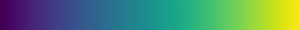cividis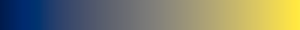magma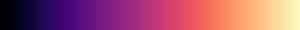inferno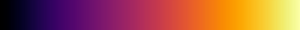plasma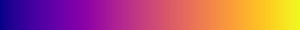parula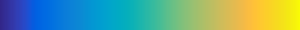heat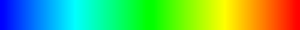hot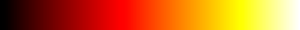jet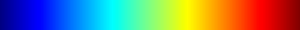gray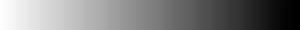github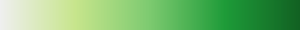Alternatively, a custom color map can be provided as custom(<color1>,<color2>,...). Neper’s legacy color map (version $$< 4$$) is custom(blue,cyan,yellow,green) and can also be obtained using legacy:

 legacy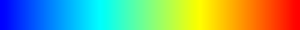Finally, it is possible to gradually fade the start of a color map, to make it starts with white. This can be done using the fade modifier, following the syntax <colormap>:fade[(threshold)]. The threshold ranges from 0 to 1 and is equal to 0.1 by default. Fading is applied linearly from 0 (full fading) to the threshold (no fading). Examples are show below:

 viridis:fade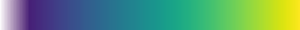viridis:fade(0.2)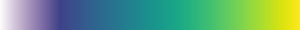1

Taken from the muparser documentation

2

Applies only to a 3D tessellation and relevant for multiscale tessellations. The scale of a face is the scale at which the face was created, and it ranges from 0 (for domain faces) to the number of scales of the tessellation (for the last created faces).

3(1,2,3)

Equivalent diameter = diameter of the circle of equivalent area/volume in 2D/3D

4

Sphericity of a polyhedron = ratio of the surface area of the sphere of equivalent volume to the surface area of the polyhedron

5

Circularity of a polygon = ratio of the perimeter of the circle of equivalent area to the perimeter of the polygon

6(1,2)

Convexity of a polyhedron (face) = ratio of the volume (surface area) of the polyhedron (face) to the volume (surface area) of the convex hull of the polyhedron (face)

7

Grain orientation spread = average disorientation (angle) with respect to the average orientation

8

A face equation is specified by the parameters $$d$$, $$a$$, $$b$$ and $$c$$, with the equation being: $$a x + b y + c z = d$$. The vector $$(a, b, c)$$ is pointing outwards of the polyhedron.

9

If a polyhedron has no neighbor on a face, a negative value is returned.

10

In the case of a lamellar tessellation with several lamella widths, lam stands for the actual lamella width of the cell (starting from 1).

11

In the case of a multimodal tessellation (e.g.in terms of cell size), mode stands for the actual mode (starting from 1). Otherwise, it is equal to 1.

12(1,2,3)

R Quey, JH Driver, PR Dawson, Intra-grain orientation distributions in hot-deformed aluminium: orientation dependence and relation to deformation mechanisms, Journal of the Mechanics and Physics of Solids, vol. 84, pp. 506-524, 2015.

13

For Gaussian distributions, they are equal to 1.349 times the values of oridisanisoangles.

14

Number of iterations during which the objective function does not decrease

15

0 if on a domain vertex, 1 if on a domain edge and 2 if on a domain face

16

Applies to a mesh of Rodrigues space

17

Applies to a mesh of the stereographic triangle

18(1,2)

1 if polygonize the circular part of a cylindrical domain and 0 otherwise.

19